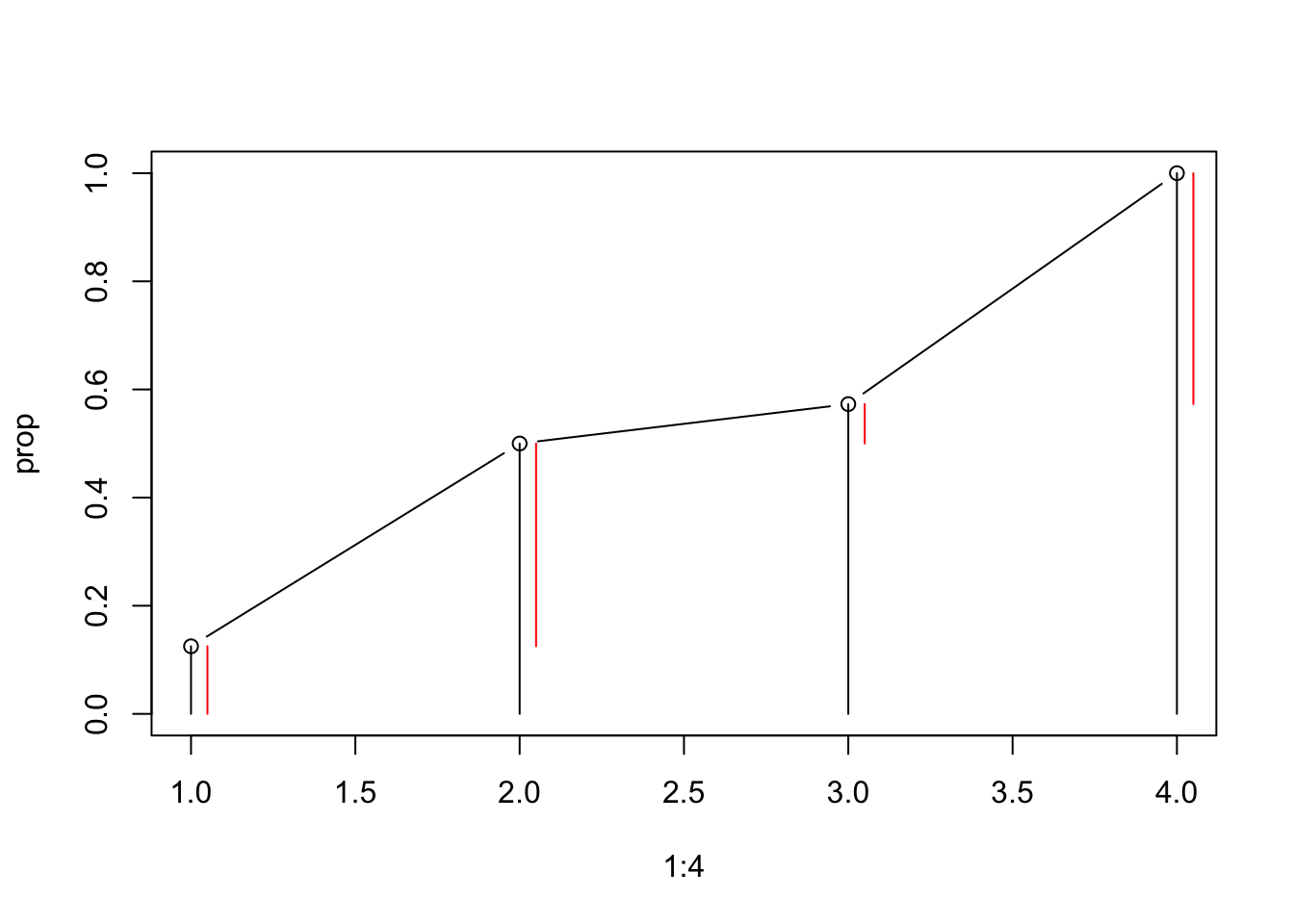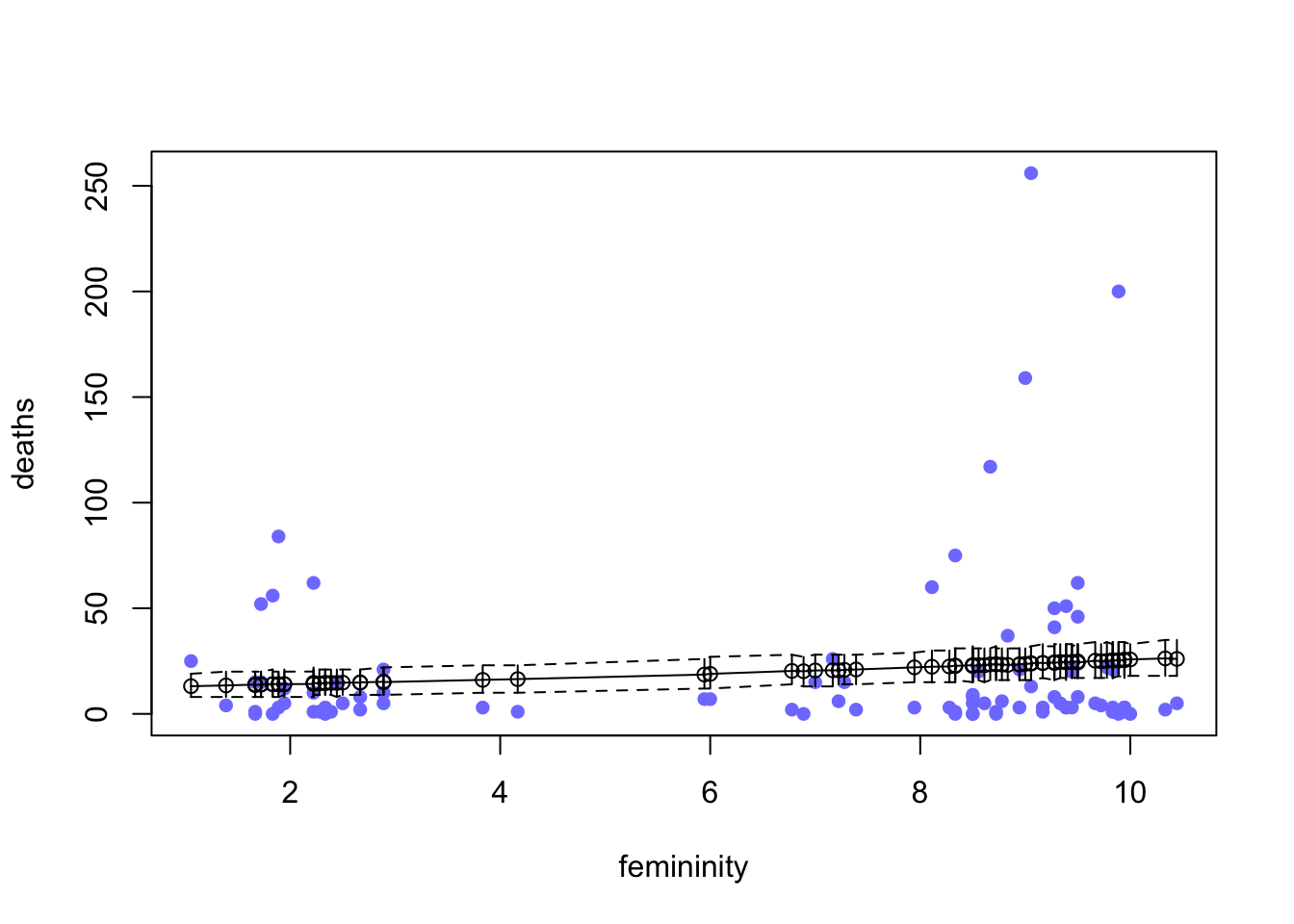## Chapter 12 - Monsters and Mixtures

This chapter introduced several new types of regression, all of which are generalizations of generalized linear models (GLMs). Ordered logistic models are useful for categorical outcomes with a strict ordering. They are built by attaching a cumulative link function to a categorical outcome distribution. Zero-inflated models mix together two different outcome distributions, allowing us to model outcomes with an excess of zeros. Models for overdispersion, such as beta-binomial and gamma-Poisson, draw the expected value of each observation from a distribution that changes shape as a function of a linear model.

Place each answer inside the code chunk (grey box). The code chunks should contain a text response or a code that completes/answers the question or activity requested. Make sure to include plots if the question requests them. Problems are labeled Easy (E), Medium (M), and Hard(H).

Finally, upon completion, name your final output .html file as: YourName_ANLY505-Year-Semester.html and publish the assignment to your R Pubs account and submit the link to Canvas. Each question is worth 5 points.

## Questions

12E1. What is the difference between an ordered categorical variable and an unordered one? Define and then give an example of each.

#Ordered categorical variables define values that represent rank or order., whereas in unordered ones, the order or rank doesn't matter. E.G.- Ordered categorical variables could be ranking number, unordered categorical variables could be marital status.

12E2. What kind of link function does an ordered logistic regression employ? How does it differ from an ordinary logit link?

# It employs the ordered logit function. It differs from an ordinary logit link by being a log-cumulative-odds link probability model. As we know, the ordered logit model is a regression model for an ordinal response variable and is based on the cumulative probabilities of the response variable instead of a discrete probability of a single event.

12E3. When count data are zero-inflated, using a model that ignores zero-inflation will tend to induce which kind of inferential error?

#In this case, there are more often zeroes than it actually has. Thus it will result in the error with less mean due to more zeros.

12E4. Over-dispersion is common in count data. Give an example of a natural process that might produce over-dispersed counts. Can you also give an example of a process that might produce underdispersed counts?

# Overdispersion is when changes are observed to be higher than expected. Some distributions have no parameters to fit the variability of observations. For example, a normal distribution does this with parameters, which are constant in a typical regression.

12M1. At a certain university, employees are annually rated from 1 to 4 on their productivity, with 1 being least productive and 4 most productive. In a certain department at this certain university in a certain year, the numbers of employees receiving each rating were (from 1 to 4): 12, 36, 7, 41. Compute the log cumulative odds of each rating.

employees <- c(12,36,7,41,0)

for(i in 1:4){
print(log(sum(employees[1:i])/sum(employees[(i+1):5])))
}
##  -1.94591
##  0
##  0.2937611
##  Inf

12M2. Make a version of Figure 12.5 for the employee ratings data given just above.

prop= employees/sum(employees)

prop=sapply(1:4,function(i){sum(prop[1:i])})
plot(y=prop,x=1:4,type="b", ylim=c(0,1))
segments(1:4,0,1:4,prop)
for(i in 1:4){segments(i+0.05,c(0,prop)[i],i+0.05,prop[i], col = "red")}12M3. Can you modify the derivation of the zero-inflated Poisson distribution (ZIPoisson) from the chapter to construct a zero-inflated binomial distribution?

#Zero-inflated binomial distribution:
#Proability of zero distribution: Pr(0|p0, q, n) = p0 + (1-p0) * (1-q) ^ n
#Probability of non-zero distribution Pr(y|p0, q, n) = (1-p0) (n!/(y!(n-y)!))(q^y)((1-q)^(n-y))

12H1. In 2014, a paper was published that was entitled “Female hurricanes are deadlier than male hurricanes.”191 As the title suggests, the paper claimed that hurricanes with female names have caused greater loss of life, and the explanation given is that people unconsciously rate female hurricanes as less dangerous and so are less likely to evacuate. Statisticians severely criticized the paper after publication. Here, you’ll explore the complete data used in the paper and consider the hypothesis that hurricanes with female names are deadlier. Load the data with:

data(Hurricanes)
m11H1.map <- map(
alist(
deaths ~ dpois( lambda ),
log(lambda) <- a + bF*femininity,
a ~ dnorm(0,10),
bF ~ dnorm(0,5)
) ,
data=Hurricanes)

m11H1.map2 <- map(
alist(
deaths ~ dpois( lambda ),
log(lambda) <- a ,
a ~ dnorm(0,10)
) ,
data=Hurricanes)

compare(m11H1.map,m11H1.map2)
##                WAIC       SE    dWAIC      dSE     pWAIC       weight
## m11H1.map  4420.795 1002.850  0.00000       NA 130.81706 9.999446e-01
## m11H1.map2 4440.395 1069.997 19.60002 136.9368  80.11385 5.544785e-05
y <- sim(m11H1.map)

y.mean <- colMeans(y)
y.PI <- apply(y, 2, PI)

plot(y=Hurricanes$deaths, x=Hurricanes$femininity, col=rangi2, ylab="deaths", xlab="femininity", pch=16)
points(y=y.mean, x=Hurricanes$femininity, pch=1) segments(x0=Hurricanes$femininity, x1= Hurricanes$femininity, y0=y.PI[1,], y1=y.PI[2,]) lines(y= y.mean[order(Hurricanes$femininity)],  x=sort(Hurricanes$femininity)) lines( y.PI[1,order(Hurricanes$femininity)],  x=sort(Hurricanes$femininity), lty=2 ) lines( y.PI[2,order(Hurricanes$femininity)],  x=sort(Hurricanes\$femininity), lty=2 )#From the plot there are some overdispersion. When we compare femininity and deaths, we can see that femninity of names is much higher when compared to femininity of deaths. Femininity of names retrodict well and Poisson model fits poorly.

Acquaint yourself with the columns by inspecting the help ?Hurricanes. In this problem, you’ll focus on predicting deaths using femininity of each hurricane’s name. Fit and interpret the simplest possible model, a Poisson model of deaths using femininity as a predictor. You can use quap or ulam. Plot your results of prior predictive simulation. Compare the model to an intercept-only Poisson model of deaths. Plot the results over the raw data. How strong is the association between femininity of name and deaths? Which storms does the model fit (retrodict) well? Which storms does it fit poorly?